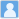• ### Neutrino Oscillation Probabilities in Matter with Direct and Indirect Unitarity Violation in the

分类： 物理学 >> 核物理学 提交时间： 2016-09-14Yu-Feng Li Shu Luo

摘要：In the presence of both direct and indirect unitarity violation in the lepton mixing matrix, we derive a complete set of series expansion formulas for neutrino oscillation probabilities in matter of constant density. Expansions in the mass hierarchy parameter α≡Δm221/Δm231 and those unitarity violation parameters s2ij (for i = 1, 2, 3 and j = 4, 5, 6) up to the first order are studied in this paper. We analyse the accuracy of the analytical series expansion formulas in different regions of L / E. A detailed numerical analysis is also performed, of which the different effects of the direct and the indirect unitarity violation are particularly emphasized. We also study in this paper the summed να→νe,ν,τprobabilities, whose deviation from the unity provides a definite signal of the unitarity violation.

同行评议状态:待评议

• ### Model Independent Approach of the JUNO $^8$B Solar Neutrino Program

分类： 天文学 >> 天文学 提交时间： 2023-02-19

摘要：The physics potential of detecting $^8$B solar neutrinos is exploited at the Jiangmen Underground Neutrino Observatory (JUNO), in a model independent manner by using three distinct channels of the charged-current (CC), neutral-current (NC) and elastic scattering (ES) interactions. Due to the largest-ever mass of $^{13}$C nuclei in the liquid-scintillator detectors and the potential low background level, $^8$B solar neutrinos would be observable in the CC and NC interactions on $^{13}$C for the first time. By virtue of optimized event selections and muon veto strategies, backgrounds from the accidental coincidence, muon-induced isotopes, and external backgrounds can be greatly suppressed. Excellent signal-to-background ratios can be achieved in the CC, NC and ES channels to guarantee the $^8$B solar neutrino observation. From the sensitivity studies performed in this work, we show that one can reach the precision levels of 5%, 8% and 20% for the $^8$B neutrino flux, $\sin^2\theta_{12}$, and $\Delta m^2_{21}$, respectively, using ten years of JUNO data. It would be unique and helpful to probe the details of both solar physics and neutrino physics. In addition, when combined with SNO, the world-best precision of 3% is expected for the $^8$B neutrino flux measurement.

同行评议状态:待评议